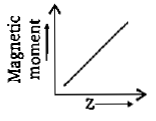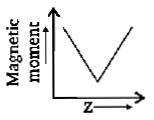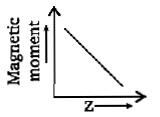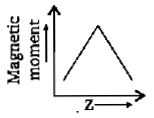The period number and group number of "Tantalum" (Z=73) are respectively :

(a) 5, 7                                                 (b)6, 13

(c)6, 5                                                  (d)None of the these

Concept Questions :-

Modern Periodic Table
High Yielding Test Series + Question Bank - NEET 2020

Difficulty Level:

Consider the following electronic configuration of an element (P) :

$\left[\mathrm{Xe}\right]4{f}^{14}5{d}^{1}6{s}^{2}$

Then correct statement about element 'P' is :

(a) It belongs to 6th period and the 1st group

(b) It belongs to 6th period and the 2nd group

(c) It belongs to 6th period and the 3rd group

(d) none of these

Concept Questions :-

Electronic Configuration
High Yielding Test Series + Question Bank - NEET 2020

Difficulty Level:

Which of the following metal is highest electropositive (metallic) in nature ?

(a) Be                                       (b) Rb

(c) Mn                                       (d) Tl

Concept Questions :-

Modern Periodic Table
High Yielding Test Series + Question Bank - NEET 2020

Difficulty Level:

Which of the following species must have maximum number of electrons in $\text{'}{d}_{xy}\text{'}$ orbital ?

(a) Cr

(b) $F{e}^{3+}$

(c) $C{u}^{+}$

(d) Both (a) and (b)

Concept Questions :-

Electronic Configuration
High Yielding Test Series + Question Bank - NEET 2020

Difficulty Level:

Which of the following graph is correct representative between atomic number (Z) and magnetic moment of d-block elements? [Outer electronic configuration :

(a)(b)(c)(d)Concept Questions :-

Electronic Configuration
High Yielding Test Series + Question Bank - NEET 2020

Difficulty Level:

If IUPAC name of an element is "unununium" then correct statement regarding element is :

(a) It is a inner transition element

(b) It belongs to 8th period in periodic table

(c) It is transition element

(d) It is a non-transition element

Concept Questions :-

Modern Periodic Table
High Yielding Test Series + Question Bank - NEET 2020

Difficulty Level:

Which property decreases from left to right across the periodic table and increases from top to bottom?

(i) Atomic radius (ii) Electronegativity (iii) Ionisation energy (iv) Metallic character

(a) (i) only                     (b) (i),(ii) and (iii)

(c) (i), (iii) and (iv)         (d) (i) and (iv)

Concept Questions :-

Modern Periodic Table
High Yielding Test Series + Question Bank - NEET 2020

Difficulty Level:

Consider the following information about element P and Q :

Period number            Group number

P                               2                                 15

Q                               3                                 2

Then formula of the compound formed by P and Q element is :

(a) PQ          (b) ${P}_{3}{Q}_{2}$            (c) ${P}_{2}{Q}_{3}$            (d)$P{Q}_{2}$

Concept Questions :-

Nature of Compound
High Yielding Test Series + Question Bank - NEET 2020

Difficulty Level:

Which electronic configuration must represent an atom in excited state?

Concept Questions :-

Electronic Configuration
High Yielding Test Series + Question Bank - NEET 2020

Difficulty Level:

Incorrect order of radius is :

(a)     (b) $\mathrm{Co}>\mathrm{Ni}>\mathrm{Cu}>\mathrm{Zn}$

(c)     (d) $A{s}^{3-}>S{e}^{2-}>C{s}^{+}>B{a}^{2}+$

Concept Questions :-

Atomic Size